Three sides of a quadrilateral are equal to the foreside which is 16 cm long. What is the length of the one with equal sides if the perimeter is 58 cm?

b =  10 cm

### Step-by-step explanation: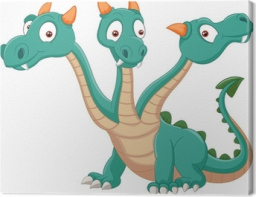Did you find an error or inaccuracy? Feel free to write us. Thank you!#### You need to know the following knowledge to solve this word math problem:

We encourage you to watch this tutorial video on this math problem:

## Related math problems and questions: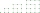The perimeter of a quadrilateral (four sided plane shape) is 125 cm . If the three known sides measure a=12 cm, b=45 cm, and c=30.5 cm, how long is the fourth side?
• The perimeter 6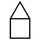The perimeter of quadrilateral is 442.95 cm. If the three known sides measure 84.56 cm,132.77 cm, and 82.75 cm, how long is the fourth side?
• Medians in triangle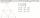Median of isosceles triangle has a length 3 cm. Determine the length of its sides if its perimeter is 16 cm.
• RectangleThe length of the rectangle is 12 cm greater than 3 times its width. What dimensions and area this rectangle has if ts circumference is 104 cm.
• Rhombus A2p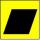Rhombus area is 13 cm2 and its height is 5cm long . Determine a perimeter of this rhombus.
• Similar trianglesTriangle A'B'C 'is similar to triangle ABC, whose sides are 5 cm, 8 cm, and 7 cm long. What is the length of the sides of the triangle A'B'C' if its circumference is 80 cm?
• The ropeA 68-centimeter long rope is used to make a rhombus on the ground. The distance between a pair of opposite side corners is 16 centimeters. What is the distance between the other two corners?
• A squareA square with a length of diagonals 12cm give: a) Calculate the area of a square b) rhombus with the same area as the square, has one diagonal with a length of 16 cm. Calculate the length of the other diagonal.
• Prism height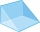What is the prism's height with the base of a right triangle of 6 cm and 9 cm? The diaphragm is 10.8 cm long. The volume of the prism is 58 cm3. Calculate its surface.
• MidpointsTriangle ABC with sides a = 5 cm, b = 3 cm, c = 40mm has a midpoint of K, L, M. How many centimeters is long perimeter of parallelogram KBLM?
• Rectangular triangle PQRIn the rectangular triangle PQR, the PQ leg is divided by the X point into two segments of which longer is 25cm long. The second leg PR has a length 16 cm. The length of the RX is 20 cm. Calculate the length p of side RQ. The result is round to 2 decimal
• HypotenuseCalculate the length of the hypotenuse of a right triangle if the length of one leg is 4 cm and its content area is 16 square centimeters.
• RhombusIf we increase the rhombus side by 2.4 cm; its perimeter is 20 cm. How long was the side of the rhombus?
• Rectangle - desc circle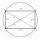Length of the sides of the rectangle are at a ratio 1: 3 . Radius of the circle circumscribed to rectangle is 10 cm. Calculate the rectangle's perimeter.
• Isosceles trapezoidFind the area of an isosceles trapezoid, if the bases are 12 cm and 20 cm, the length of the arm is 16 cm
• The perimeter 3The perimeter of a rectangle is 35 cm. The ratio of the length to its width is 3:2. Calculate the dimensions of the rectangle
• A perimeter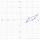A perimeter of a parallelogram is 2.8 meters. The length of one of its sides is equal to one-seventh of the entire perimeter. Find lengths of the sides of the parallelogram.